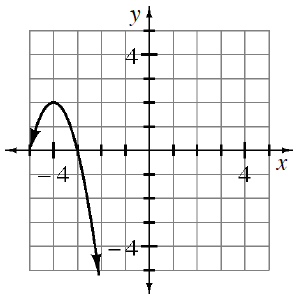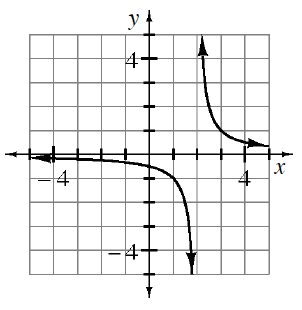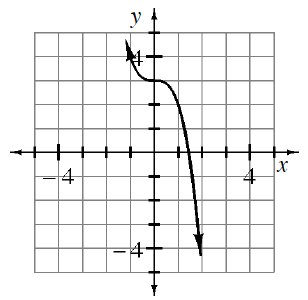### Home > CCA2 > Chapter 6 > Lesson 6.1.2 > Problem6-23

6-23.

Find an equation that will generate each graph.

1.What kind of graph is this?

What are the $x$-intercepts and the vertex?

Use the vertex to write equation in graphing form.
Then substitute one of the $x$-intercepts in for $x$ and $y$ to solve for $a$.
$y=a(x+4)^2+2$

1.The parent graph is $\text{ }y=\frac{1}{x}$ and the locator point is $(2,0)$.

1.See part (a).